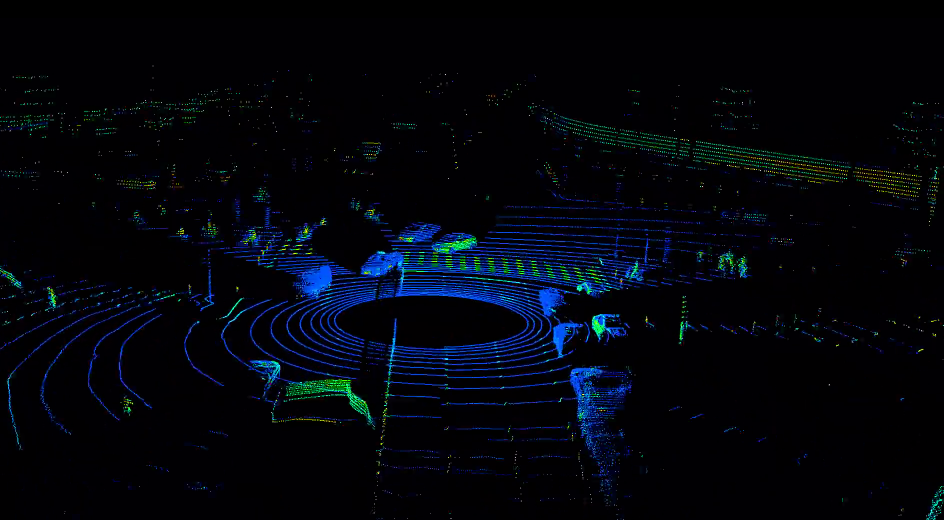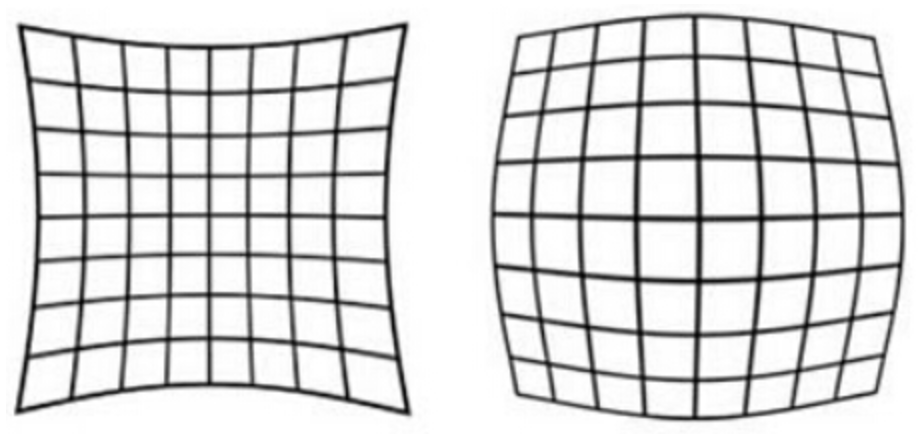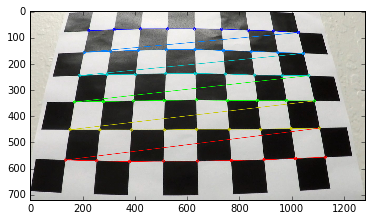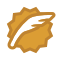# 无人驾驶汽车系统入门（六）——基于传统计算机视觉的车道线检测(1)

## 视觉感知VS激光雷达## 相机标定1. 切向畸变（tangential distortion）：是由于透镜本身与相机传感器平面（成像平面）或图像平面不平行而产生的，这种情况多是由于透镜被粘贴到镜头模组上的安装偏差导致。

xcorr=xdis(1+k1r2+k2r4+k3r6)

ycorr=ydis(1+k1r2+k2r4+k3r6)

xcorr=xdis+[2p1xdisydis+p2(r2+2x2dis)]

ycorr=ydis+[p1(r2+2y2dis)+2p2xdisydis]

• xdis$x_{dis}$ydis$y_{dis}$ 表示有畸变的坐标；
• xcorr$x_{corr}$ycorr$y_{corr}$ 表示修复后的坐标；
• k1$k_1$k2$k_2$k3$k_3$ 表示径向畸变参数；
• p1$p_1$p2$p_2$ 表示切向畸变参数；
• r$r$ 表示矫正以后的坐标到图片中心的距离#### 相机，棋盘图#### 使用OpenCV找出棋盘的对角点

from __future__ import print_function

import numpy as np
import cv2
import matplotlib.pyplot as plt
import pickle
%matplotlib inline

fig1 = plt.figure(1, figsize=(16, 9))
cal_gray = cv2.cvtColor(cal, cv2.COLOR_RGB2GRAY)
plt.subplot(2,2,1)
plt.imshow(cal)
plt.subplot(2,2,2)
plt.imshow(cal_gray, cmap='gray')ret, corners = cv2.findChessboardCorners(cal_gray, (9, 6),None)
if ret == True:
cal = cv2.drawChessboardCorners(cal, (9, 6), corners, ret)
plt.imshow(cal)objp = np.zeros((6*9, 3), np.float32)
objp[:, :2] = np.mgrid[0:9, 0:6].T.reshape(-1, 2)

img_points = []
obj_points = []

img_points.append(corners)
obj_points.append(objp)

image_size = (cal.shape, cal.shape)
ret, mtx, dist, rvecs, tvecs = cv2.calibrateCamera(obj_points, img_points,
image_size, None, None)

# Read in a test image
undist = cv2.undistort(img, mtx, dist, None, mtx)
plt.subplot(2,2,1)
plt.imshow(img)
plt.subplot(2,2,2)
plt.imshow(undist)## 确定ROI

ROI(Region of interest) 即我们处理一个视觉任务时“感兴趣的区域”，当然不同的任务ROI是不一样的，对于车道线检测而言（如下图），ROI就是车辆的前方的车道线区域：def perspective_transform(img, M):
img_size = (img.shape, img.shape)
warped = cv2.warpPerspective(img, M, img_size, flags=cv2.INTER_LINEAR)
return warped

# left_top to left_bottom,
corners = [(603, 445), (677, 445), (1105, 720), (205, 720)]

wrap_offset = 150
src_corners = [(603, 445), (677, 445), (1105, 720), (205, 720)]
dst_corners = [(205 + wrap_offset, 0), (1105 - wrap_offset, 0), (1105 - wrap_offset, 720), (205 + wrap_offset, 720)]
M = cv2.getPerspectiveTransform(np.float32(src_corners), np.float32(dst_corners))
wrap_img= perspective_transform(straight_lines1, M)

subplot(1, 2, [straight_lines1, wrap_img])AdamShanCSDN认证博客专家 图像处理 深度学习 TensorFlow

#### 计算机视觉的七大应用案例

07-2212-291万+

#### 无人驾驶汽车系统入门（八）——机器学习入门

05-292万+

#### 无人驾驶汽车系统入门（十七）——无人驾驶系统基本框架

05-261万+

#### 无人驾驶汽车系统入门（三十）——基于深度神经网络LaneNet的车道线检测及ROS实现

05-09453

#### 让车辆“学会”识别车道：使用计算机视觉（传统CV）进行车道检测

06-032万+

#### 无人驾驶汽车系统入门（十八）——使用pure pursuit实现无人车轨迹追踪

11-113970

#### 无人驾驶（基于计算机视觉的高精度地图）

08-133万+

#### 无人驾驶--车道线检测实战（附源码）

09-291万+

#### 无人驾驶汽车系统入门（二十四）——激光雷达的地面-非地面分割和pcl_ros实践

05-282639

#### 自动驾驶之无人驾驶车辆与模型预测控制©️2020 CSDN 皮肤主题: 大白 设计师: CSDN官方博客点击重新获取扫码支付1.余额是钱包充值的虚拟货币，按照1:1的比例进行支付金额的抵扣。
2.余额无法直接购买下载，可以购买VIP、C币套餐、付费专栏及课程。余额充值